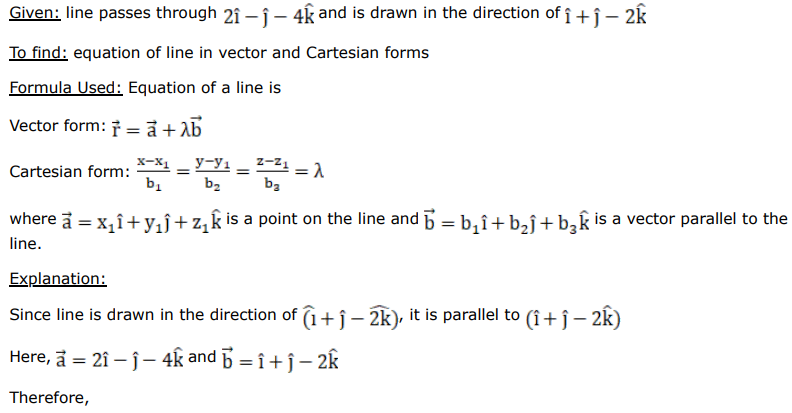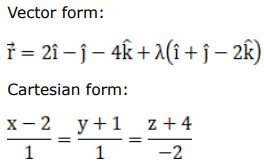# A line is drawn in the direction ofQuestion:

A line is drawn in the direction of $(\hat{\mathrm{i}}+\hat{\mathrm{j}}-2 \hat{\mathrm{k}})$ and it passes through a point with position vector $(2 \hat{\mathrm{i}}-\hat{\mathrm{j}}-4 \hat{\mathrm{k}}) .$ Find the equations of the line in the vector as well as Cartesian forms.

Solution: